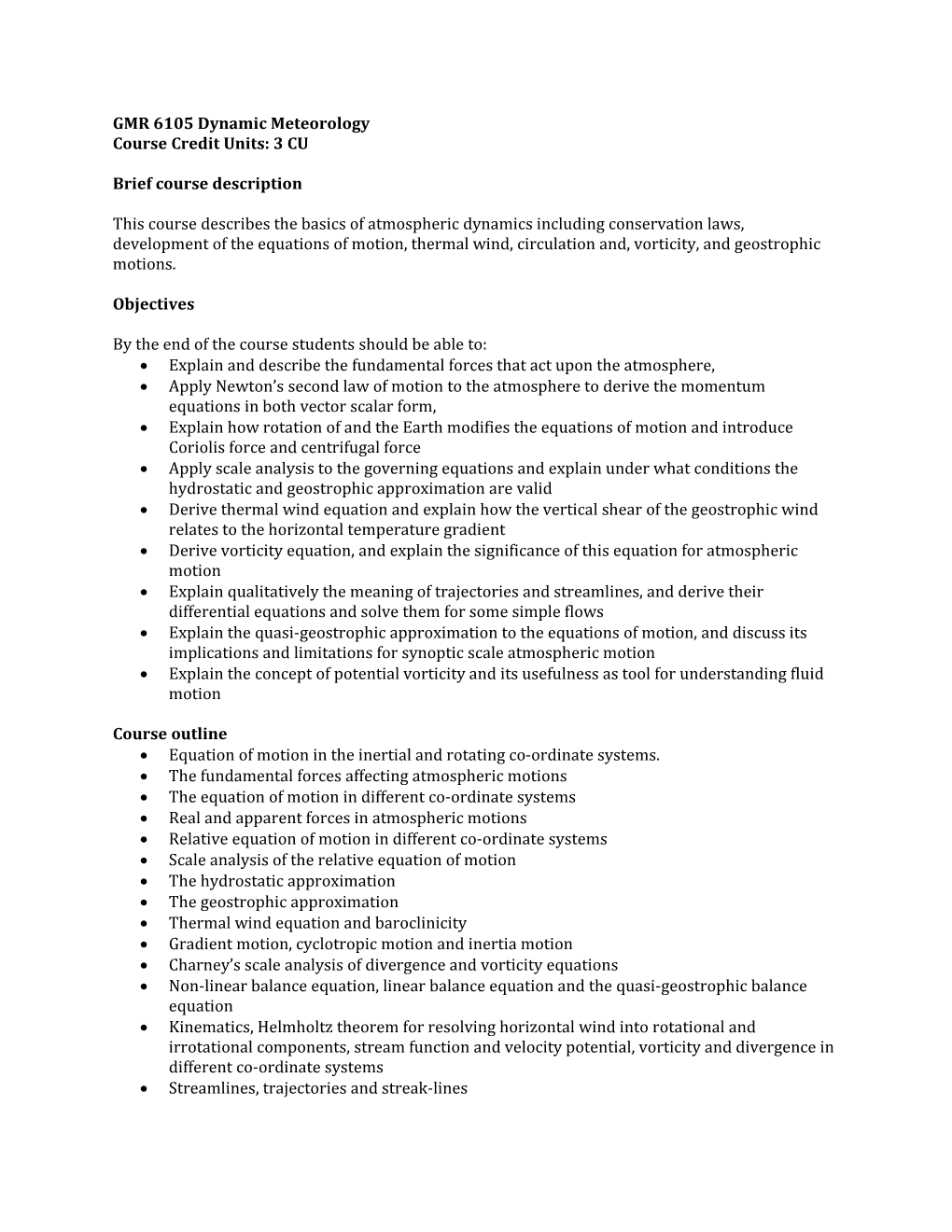# GMR 6105 Dynamic MeteorologyGMR 6105 Dynamic Meteorology

Course Credit Units: 3 CU

Brief course description

This course describes the basics of atmospheric dynamics including conservation laws, development of the equations of motion, thermal wind, circulation and, vorticity, and geostrophic motions.

Objectives

By the end of the course students should be able to:

• Explain and describe the fundamental forces that act upon the atmosphere,
• Apply Newton’s second law of motion to the atmosphere to derive the momentum equations in both vector scalar form,
• Explain how rotation of and the Earth modifies the equations of motion and introduce Coriolis force and centrifugal force
• Apply scale analysis to the governing equations and explain under what conditions the hydrostatic and geostrophic approximation are valid
• Derive thermal wind equation and explain how the vertical shear of the geostrophic wind relates to the horizontal temperature gradient
• Derive vorticity equation, and explain the significance of this equation for atmospheric motion
• Explain qualitatively the meaning of trajectories and streamlines, and derive their differential equations and solve them for some simple flows
• Explain the quasi-geostrophic approximation to the equations of motion, and discuss its implications and limitations for synoptic scale atmospheric motion
• Explain the concept of potential vorticity and its usefulness as tool for understanding fluid motion

Course outline

• Equation of motion in the inertial and rotating co-ordinate systems.
• The fundamental forces affecting atmospheric motions
• The equation of motion in different co-ordinate systems
• Real and apparent forces in atmospheric motions
• Relative equation of motion in different co-ordinate systems
• Scale analysis of the relative equation of motion
• The hydrostatic approximation
• The geostrophic approximation
• Thermal wind equation and baroclinicity
• Gradient motion, cyclotropic motion and inertia motion
• Charney’s scale analysis of divergence and vorticity equations
• Non-linear balance equation, linear balance equation and the quasi-geostrophic balance equation
• Kinematics, Helmholtz theorem for resolving horizontal wind into rotational and irrotational components, stream function and velocity potential, vorticity and divergence in different co-ordinate systems
• Streamlines, trajectories and streak-lines

Mode of Delivery: Lectures, tutorials and assignments

Assessment: Assignments and tests (30%), Examination (70%), total (100%)